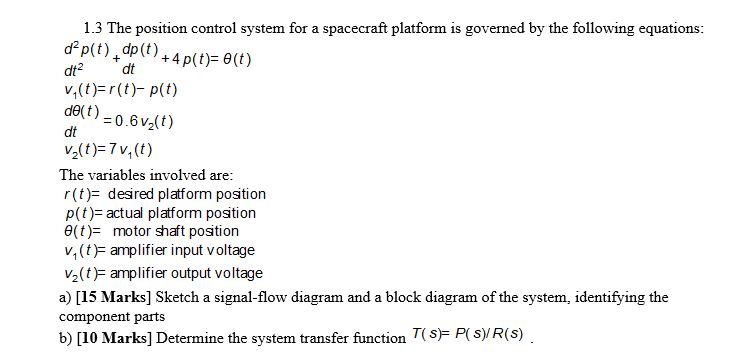### Create an Account

Home / Questions / 1.3 The position control system for a spacecraft platform is governed by the following equations d2

# 1.3 The position control system for a spacecraft platform is governed by the following equations d21.3 The position control system for a spacecraft platform is governed by the following equations d2 p(t dp 4 p(t)= 0(t) + + dt2 dt v,(t)r(t)p(t) de(t)0.6v2(t) dt v2(t)7v, (t) The variables involved are r(t) desired platform position p(t) actual platform position (t) motor shaft position v, (t) amplifier input voltage v2(t)amplifier output voltage a) [15 Marks] Sketch a signal-flow diagram and a block diagram of the system, identifying the component parts b) [10 Marks] Determine the system transfer function T(s)= P(s)/R(s)

Apr 06 2020 View more View LessSubscribe To Get Solution